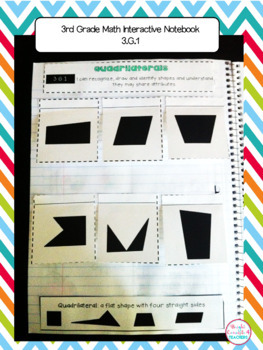3rd Grade Math Interactive Notebook {Common Core Aligned}3rd, Homeschool
Subjects
Standards
Resource Type
Formats Included
• PDF
Pages
120 pages

Also included in

1. Save with this bundle of THIRD Grade ELA & MATH Interactive Notebooks! This bundle includes the following 3 notebooks from my store:Math Interactive NotebookLanguage Interactive NotebookReading Interactive Notebook**If you purchase each notebook separately, it would cost \$38.00. You are getting
\$32.00
\$38.00
Save \$6.00

Description

Interactive notebooks are a great learning tool in the classroom. They serve as a resource for reviewing standards, a reference tool, or even as an assessment.

This notebook covers all the 3rd Grade Common Core MATH standards (including Operations & Algebraic Thinking, Numbers and Operations in Base 10, Geometry, Measurement and Data, and Number and Operations-Fractions )

This product includes:

-Notebook cover

-Divider tabs

-Strand Dividers

-Pictures of EVERY page so you can have an example of how each activity can be used

-Student friendly "I can" statements at the top of each page.

I know your students will love making these notebooks as a method to reinforce all the CCSS math standards they have been taught.

Skills covered:

Operations and Algebraic Thinking:

-Understanding Multiplication with pictures

-Quotients

-Problem Solving with Multiplication and Missing Factors

-Problem Solving with Division

-Problem Solving with Division and Three Digit Numbers

-Multiplication and Division with an unknown number

-Properties of Multiplication

-Fact Families with Multiplication and Division

-Understanding Division

-Multiplication Chart

-Division Chart

-Multi-step Word Problems

-Using Variable Equations to solve word problems

-Rounding

-Estimation to solve word problems

-Addition, Subtraction, Multiplication and Division pattern tables

Numbers and Operations in Base 10:

-Place Value with Rounding

-Word Problem

-Multiplying one digit numbers by multiples of 10

Geometry:

-Solid Figures

-Plane Shapes

-Equal Parts

-Fractions

Measurement and Data:

-Telling Time

-Elapse Time

-Time Word Problems

-Time Lines

-Metric Measurement and Word Problem

-Bar Graphs

-Pictographs

-Measuring and Line Plots

-Area and Square Units

-Area of a rectangle

-Area word problems

-Perimeter

-Area v. Perimeter word problem

Number and Operations-Fractions

-Identifying Fractions

-Fractions on a Number line

-Equivalent Fractions

-Reducing Fractions

-Ordering Fractions

-Whole Numbers and Fractions

-Mixed Numbers

-Comparing Fractions

-Word Problems

3rd Grade Interactive Notebook BUNDLE {ELA & MATH}

3rd Grade ELA Interactive Notebook BUNDLE

More Math Interactive Notebooks:

Kindergarten Math Interactive Notebook

***************************************************

If you would like to get updates on NEW & CURRENT resources...

**************************************************

Email me at brightconcepts4teachers@gmail.com if you have any questions!

Total Pages
120 pages
N/A
Teaching Duration
1 Year
Report this Resource to TpT
Reported resources will be reviewed by our team. Report this resource to let us know if this resource violates TpT’s content guidelines.

Standards

to see state-specific standards (only available in the US).
Partition shapes into parts with equal areas. Express the area of each part as a unit fraction of the whole. For example, partition a shape into 4 parts with equal area, and describe the area of each part as 1/4 of the area of the shape.
Understand that shapes in different categories (e.g., rhombuses, rectangles, and others) may share attributes (e.g., having four sides), and that the shared attributes can define a larger category (e.g., quadrilaterals). Recognize rhombuses, rectangles, and squares as examples of quadrilaterals, and draw examples of quadrilaterals that do not belong to any of these subcategories.
Compare two fractions with the same numerator or the same denominator by reasoning about their size. Recognize that comparisons are valid only when the two fractions refer to the same whole. Record the results of comparisons with the symbols >, =, or <, and justify the conclusions, e.g., by using a visual fraction model.
Express whole numbers as fractions, and recognize fractions that are equivalent to whole numbers. Examples: Express 3 in the form 3 = 3/1; recognize that 6/1 = 6; locate 4/4 and 1 at the same point of a number line diagram.
Recognize and generate simple equivalent fractions, (e.g., 1/2 = 2/4, 4/6 = 2/3). Explain why the fractions are equivalent, e.g., by using a visual fraction model.